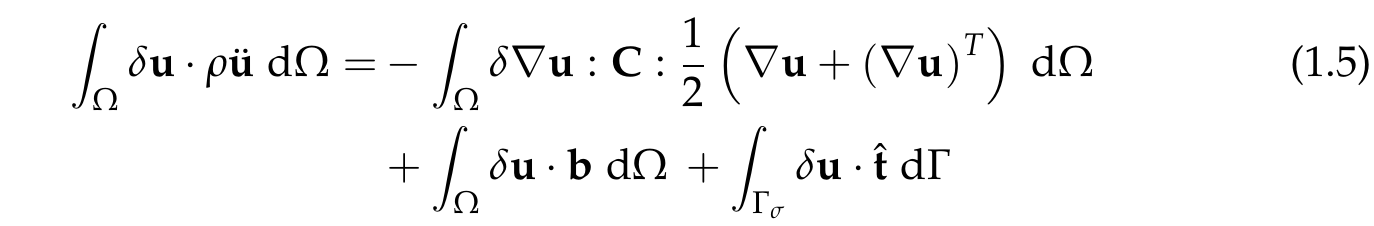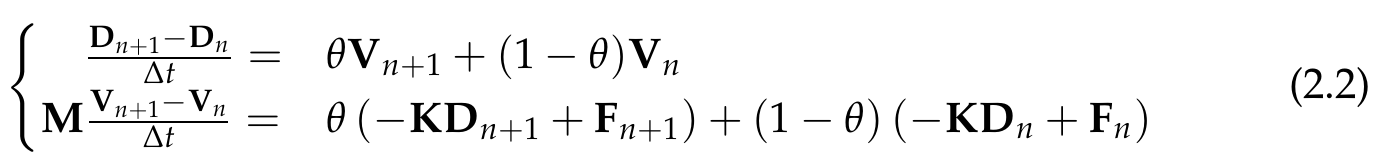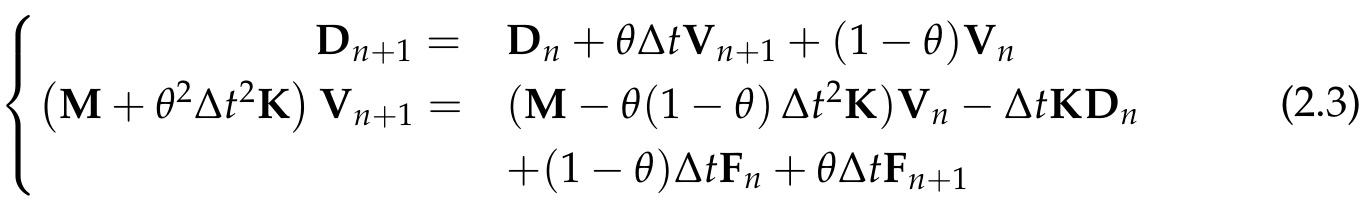Since deal.II is a library and you are free to implement your own stuff, this section provides details about the implemented solver analogous to the commented tutorial programs in deal.II. In case you want to modify this solver or use it for your own project. The linear elastic solver was built on the step-8 tutorial program of the deal.II library, which deals with linear elastostatics. The nonlinear elastic solver was built on the Quasi static Finite strain Compressible Elasticity code gallery example. A lot of aspects are already explained there and in the source code files. However, these programs deal with elastodynamics. Therefore, we need to consider a time discretization.

As a quick overview:

## Linear elastic solver

### Mathematical aspects

Our starting equation, which is basically the Navier-Cauchy equation, reads as follows:Where u is the displacement field, rho the material density, b the body forces and sigma the stress tensor, which is related to the linear strain measure epsilon via the fourth order elasticity tensor C. Equation 1.1 needs to be satisfied in the whole domain Omega and we apply the following boundary conditions:Here, the first boundary condition is applied to the Dirichlet boundary Gamma_u and we prescribe a zero displacement. The second boundary condition is applied to the Neumann boundary Gamma_sigma and describes basically our coupling interface, since the traction vector is obtained from our flow solver. As last point, the initial conditions are given in equation 1.3:Both initial values are chosen to be zero, but you are free to choose them differently according to your problem. The material is assumed as isotropic and thus fully described by the Lamé coefficients:Where 1 and I are the second and fourth order unit tensors respectively. Finally, the weak formulation of equation 1.1 is given as#### Discretization

Discretization in space is done using Finite Elements. By default, linear shape functions are applied, but you are free to specify the polynomial degree in the parameters.prm file. More details about the Finite Element discretization are available in the step-8 tutorial description (see link above). The following section focuses on the time discretization. Therefore, the governing second order differential equation is transformed, similar to a state space model, in two first order equations:Here, a block notation for the global vectors and matrices is used, where M denotes the mass matrix, K the stiffness matrix, D the displacement vector, V the velocity vector, and F the load vector, which includes body loads and the prescribed traction. Note that the load vetor F is due to the coupling time dependent. Time derivatives are approximated using a one-step theta methodwhere theta is a parameter $$[0,1]$$, which allows to modify the time stepping properties. $$\theta = 0$$ results in a forward Euler method and $$\theta = 1$$ results in a backward Euler method, which are both first order accurate. Solely $$\theta = 0.5$$ results in a second order accurate Crank-Nicolson scheme, which additionally provides energy conservation in the system.

Performing some equation massaging finally leads to the following system, which is actually implemented:Hence, we solve in each time step for the unknown velocity and update later on for the unknown displacement. Before the time loop is entered, time invariant global matrices are assembled in the assemble_system() function, namely the stiffness matrix K, the mass matrix M, and the constant body loads (gravity). Since the composition of the linear system on the left-hand side (which consists of M and K) is also constant, we store it in a stepping matrix, in order to save the rebuilding in each time step. Note that in the tutorial cases, no gravity is needed and therefore, the term is zero in the example program.

The time-dependent terms are assembled in the assemble_rhs() function in each time step. This is straightforward and comments have been added in the source code for more information. Part two of equation 2.3 is finally solved in the solve() function, before the displacement vector is updated in the update_displacement() function (part one of eq. 2.3).

## Capability

This linear solver is designed for single-core and single-thread computations. If you like to change the source code for parallel computations, have a look at the step-17 tutorial program, which shows how this can be done using PETSc.

Furthermore, this section should point out that the underlying physical description of the linear structural mechanics is not suitable for large deformations and large rotations. The reason is simply the linear measurement of strains, which are only valid for small deviations. Rigid body rotations lead already to an indicated artificial strain. Due to this, the structure usually gets bigger and a small rotation assumption is violated. Hence, a linear strain measure is typically used for rotations smaller than 6°.